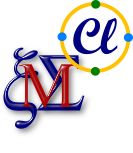Quaternion multiplication table

$$\begin{pmatrix}1 & {{e}_{1}} & {{e}_{2}} & {{e}_{1}}\mathit{ . }{{e}_{2}}\cr {{e}_{1}} & -1 & {{e}_{1}}\mathit{ . }{{e}_{2}} & -{{e}_{2}}\cr {{e}_{2}} & -{{e}_{1}}\mathit{ . }{{e}_{2}} & -1 & {{e}_{1}}\cr {{e}_{1}}\mathit{ . }{{e}_{2}} & {{e}_{2}} & -{{e}_{1}} & -1\end{pmatrix}$$

Quaternions were first described by Irish mathematician William Rowan Hamilton in 1843 for describing rotations in 3D space.  Unit quaternions, also known as versors, provide a convenient mathematical notation for representing orientations and rotations of objects in three dimensions.Later, William Clifford invented his algebras in 1876 as an attempt to generalize the quaternions to higher dimensions.  Clifford algebra unifies all branches of physics, and has found rich applications in robotics, signal processing, ray tracing, virtual reality, computer vision, vector field processing, tracking, geographic information systems and neural computing. Proponents of Geometric algebra and Geometric calculus promote the view that these approaches unify, simplify, and generalize vast areas of mathematics that involve geometric ideas. Clifford algebras provide natural generalizations of complex, dual and double numbers into the concept of Clifford numbers.

Members of Belgian INCF node support the project Clifford for the computer algebra system Maxima.Maxima is a system for the manipulation of symbolic and numerical expressions, including differentiation, integration, Taylor series, Laplace transforms, ordinary differential equations, systems of linear equations, polynomials, sets, lists, vectors, matrices and tensors. Maxima yields high precision numerical results by using exact fractions, arbitrary-precision integers and variable-precision floating-point numbers. Maxima can plot functions and data in two and three dimensions. Every Maxima expression is simultaneously also a lambda-construct and its value is the value of the last assigned member. The Maxima language is based on the ideas of functional programming, which is particularly well suited for transformations of formal mathematical expressions.